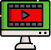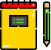VideosNotes

### Calculations Involving Multiplication & Division Using Logarithm. - 1

Calculations Involving Multiplic...
In this lesson, we will be learning, calculations involving multiplication & division using Logarithm.
Views: N/A
Video Duration: 9:28 (Part 1 of 3)